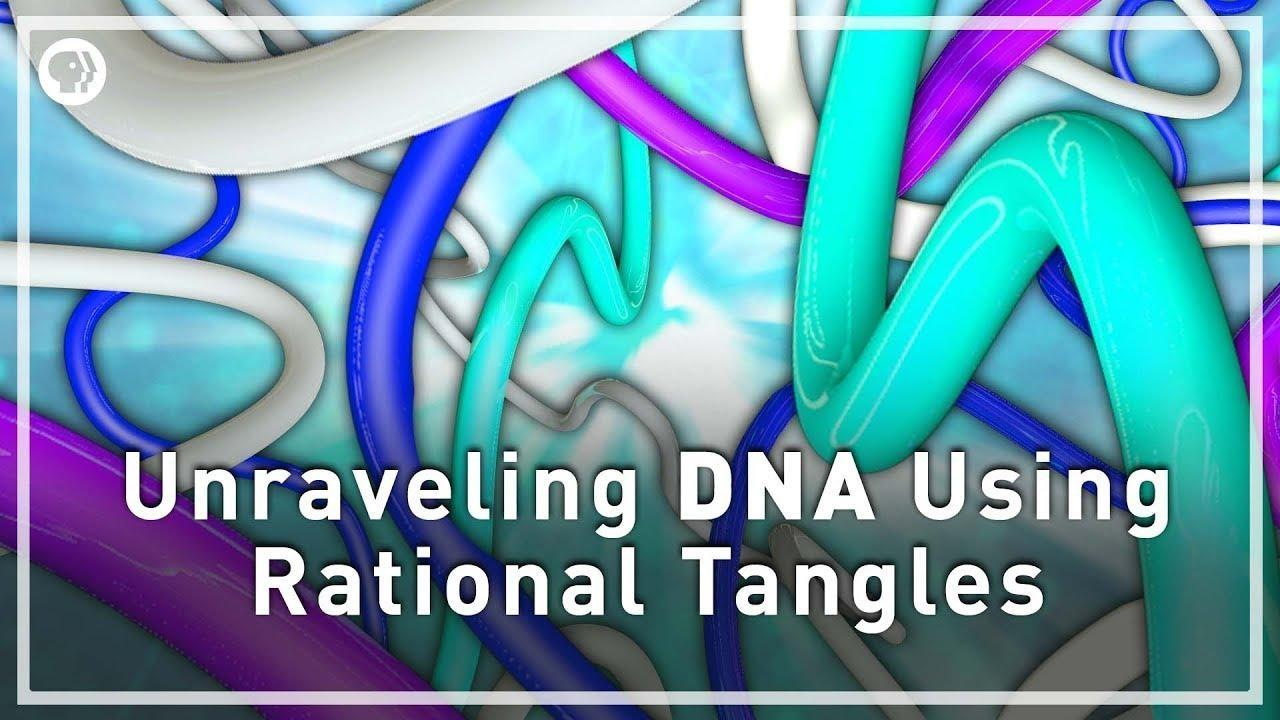# Infinite Series

Math is pervasive - a robust yet precise language - and with each episode you’ll begin to

10m 21s

#### The Geometry of SET

In the card game SET, what is the maximum number of cards you can deal that might not contain a SET?

10m 21s

#### Topology vs "a" Topology

What exactly is a topological space?

9m 35s

#### What Does It Mean to Be a Number? (The Peano Axioms)

If you needed to tell someone what numbers are and how they work, without using the notion of number in your answer, could you do it?

10m 31s

#### Defining Infinity

Set theory is supposed to be a foundation of all of mathematics. How does it handle infinity?

8m 2s

#### This Video was Not Encrypted with RSA

Here we break down Asymmetric crypto and more.

8m 16s

#### Proving Brouwer's Fixed Point Theorem

There is a proof for Brouwer's Fixed Point Theorem that uses a bridge - or portal - between geometry and algebra.

9m 32s

#### Instant Insanity Puzzle

Imagine you have four cubes, whose faces are colored red, blue, yellow, and green. Can you stack these cubes so that each color appears exactly once on each of the four sides of the stack?

8m 21s

#### Associahedra: The Shapes of Multiplication

What happens when you multiply shapes?

7m 17s

#### Telling Time on a Torus

What shape do you most associate with a standard analog clock? Your reflex answer might be a circle, but a more natural answer is actually a torus. Surprised? Then stick around.

13m 2s

#### How Big are All Infinities Combined? (Cantor's Paradox)

Infinities come in different sizes. There’s a whole tower of progressively larger "sizes of infinity". So what’s the right way to describe the size of the whole tower?

7m 28s

#### The Multiplication Multiverse

What happens if you multiply things that aren’t numbers? And what happens if that multiplication is not associative?

8m 57s

#### Beyond the Golden Ratio

You know the Golden Ratio, but what is the Silver Ratio?

9m 36s

#### Unraveling DNA with Rational Tangles

When you think about math, what do you think of? Numbers? Equations? Patterns maybe? How about… knots? As in, actual tangles and knots?

8m 1s

#### How to Divide by "Zero"

What happens when you divide things that aren’t numbers?

10m 50s

#### What was Fermat’s “Marvelous" Proof?

If Fermat had a little more room in his margin, what proof would he have written there?

9m 12s

#### The Assassin Puzzle

Imagine you have a square-shaped room, and inside there is an assassin and a target. And suppose that any shot that the assassin takes can ricochet off the walls of the room, just like a ball on a billiard table. Is it possible to position a finite number of security guards inside the square so that they block every possible shot from the assassin to the target?

9m 36s

#### The Mathematics of Diffie-Hellman Key Exchange

Symmetric keys are essential to encrypting messages. How can two people share the same key without someone else getting a hold of it? Upfront asymmetric encryption is one way, but another is Diffie-Hellman key exchange.

10m 7s

#### What are Numbers Made of?

In the physical world, many seemingly basic things turn out to be built from even more basic things. Molecules are made of atoms, atoms are made of protons, neutrons, and electrons. So what are numbers made of?

Supported by